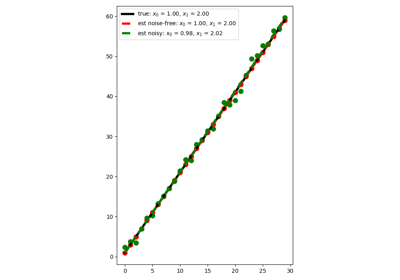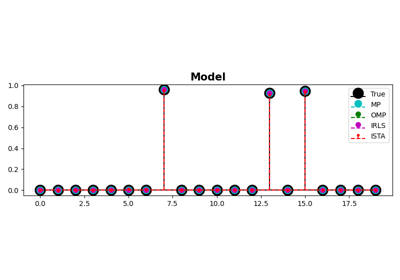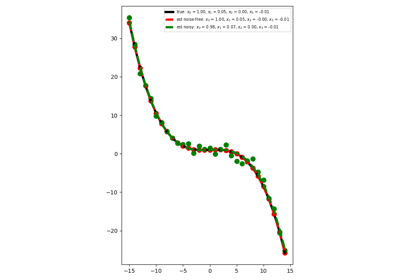# pylops.optimization.sparsity.IRLS¶

pylops.optimization.sparsity.IRLS(Op, data, nouter, threshR=False, epsR=1e-10, epsI=1e-10, x0=None, tolIRLS=1e-10, returnhistory=False, kind='data', **kwargs_solver)[source]

Iteratively reweighted least squares.

Solve an optimization problem with $$L1$$ cost function (data IRLS) or $$L1$$ regularization term (model IRLS) given the operator Op and data y.

In the data IRLS, the cost function is minimized by iteratively solving a weighted least squares problem with the weight at iteration $$i$$ being based on the data residual at iteration $$i-1$$. This IRLS solver is robust to outliers since the L1 norm given less weight to large residuals than L2 norm does.

Similarly in the model IRLS, the weight at at iteration $$i$$ is based on the model at iteration $$i-1$$. This IRLS solver inverts for a sparse model vector.

Parameters: Op : pylops.LinearOperator Operator to invert data : numpy.ndarray Data nouter : int Number of outer iterations threshR : bool, optional Apply thresholding in creation of weight (True) or damping (False) epsR : float, optional Damping to be applied to residuals for weighting term espI : float, optional Tikhonov damping x0 : numpy.ndarray, optional Initial guess tolIRLS : float, optional Tolerance. Stop outer iterations if difference between inverted model at subsequent iterations is smaller than tolIRLS returnhistory : bool, optional Return history of inverted model for each outer iteration of IRLS kind : str, optional Kind of solver (data or model) **kwargs_solver Arbitrary keyword arguments for scipy.sparse.linalg.cg solver for data IRLS and scipy.sparse.linalg.lsqr solver for model IRLS when using numpy data(or pylops.optimization.solver.cg and pylops.optimization.solver.cgls when using cupy data) xinv : numpy.ndarray Inverted model nouter : int Number of effective outer iterations xinv_hist : numpy.ndarray, optional History of inverted model rw_hist : numpy.ndarray, optional History of weights

Notes

Data IRLS solves the following optimization problem for the operator $$\mathbf{Op}$$ and the data $$\mathbf{d}$$:

$J = ||\mathbf{d} - \mathbf{Op} \mathbf{x}||_1$

by a set of outer iterations which require to repeatedly solve a weighted least squares problem of the form:

$\mathbf{x}^{(i+1)} = \operatorname*{arg\,min}_\mathbf{x} ||\mathbf{d} - \mathbf{Op} \mathbf{x}||_{2, \mathbf{R}^{(i)}}^2 + \epsilon_I^2 ||\mathbf{x}||_2^2$

where $$\mathbf{R}^{(i)}$$ is a diagonal weight matrix whose diagonal elements at iteration $$i$$ are equal to the absolute inverses of the residual vector $$\mathbf{r}^{(i)} = \mathbf{y} - \mathbf{Op} \mathbf{x}^{(i)}$$ at iteration $$i$$. More specifically the j-th element of the diagonal of $$\mathbf{R}^{(i)}$$ is

$R^{(i)}_{j,j} = \frac{1}{|r^{(i)}_j|+\epsilon_R}$

or

$R^{(i)}_{j,j} = \frac{1}{max(|r^{(i)}_j|, \epsilon_R)}$

depending on the choice threshR. In either case, $$\epsilon_R$$ is the user-defined stabilization/thresholding factor .

Similarly model IRLS solves the following optimization problem for the operator $$\mathbf{Op}$$ and the data $$\mathbf{d}$$:

$J = ||\mathbf{x}||_1 \quad s.t. \quad \mathbf{d} = \mathbf{Op} \mathbf{x}$

by a set of outer iterations which require to repeatedly solve a weighted least squares problem of the form:

$\mathbf{x}^{(i+1)} = \operatorname*{arg\,min}_\mathbf{x} ||\mathbf{x}||_{2, \mathbf{R}^{(i)}}^2 \quad s.t. \quad \mathbf{d} = \mathbf{Op} \mathbf{x}$

where $$\mathbf{R}^{(i)}$$ is a diagonal weight matrix whose diagonal elements at iteration $$i$$ are equal to the absolute inverses of the model vector $$\mathbf{x}^{(i)}$$ at iteration $$i$$. More specifically the j-th element of the diagonal of $$\mathbf{R}^{(i)}$$ is

$R^{(i)}_{j,j} = |x^{(i)}_j|$

## Examples using pylops.optimization.sparsity.IRLS¶Linear RegressionMP, OMP, ISTA and FISTAPolynomial Regression